# GMAT Math : Calculating the length of the side of a right triangle

## Example Questions

### Example Question #1 : Calculating The Length Of The Side Of A Right Triangle

What is the side length of a right triangle with a hypotenuse ofand a side of?Explanation:

We need to use the Pythagorean theorem: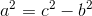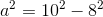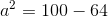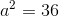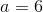### Example Question #8 : Right Triangles

There is a big square that consists of four identical right triangles and a small square. If the area of the small square is 1, the area of the big square is 5, what is the length of the shortest side of the right triangles?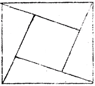Explanation:

The area of the big square is 5, and area of the small square is 1. Therefore, the area of the four right triangles is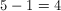.

Since the four triangles are all exactly the same, the area of each of the right triangle is 1. We know that the longer side is 2 times the shorter side, so we can represent the shorter side asand the longer side as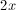. Then we are able to set up an equation: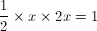Therefore, x, the length of the shortest side of the right triangle, is 1.

### Example Question #1 : Right Triangles

A triangle has sides of length 9, 12, and 16. Which of the following statements is true?

The triangle is obtuse and scalene.

The triangle cannot exist.

The triangle is acute and scalene.

The triangle is acute and isosceles.

The triangle is obtuse and isosceles.

The triangle is obtuse and scalene.

Explanation:

This triangle can exist by the Triangle Inequality, since the sum of the lengths of its shortest two sides exceeds that of the longest side: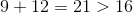The sum of the squares of its shortest two sides is less than the square of that of its longest side: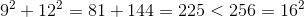This makes the triangle obtuse.

Its sides are all of different measure, which makes the triangle scalene as well.

Obtuse and scalene is the correct choice.

### Example Question #10 : Right Triangles

Using the following right traingle, calculate the value of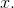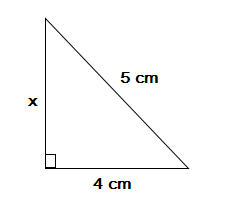(Not drawn to scale.)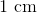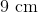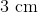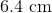Explanation:

We can determine the length of the side by using the Pythagorean Theorem: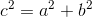where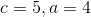Our equation is then: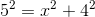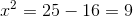### Example Question #1 : Calculating The Length Of The Side Of A Right Triangle

Calculate the length of the side of the following right triangle.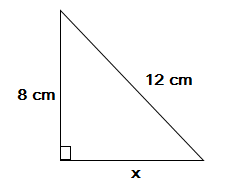(Not drawn to scale.)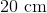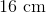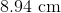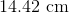Explanation:

We can calculate the length of the side by using the pythagorean theorem:where our values are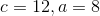we can then solve for: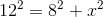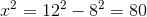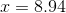### Example Question #2 : Calculating The Length Of The Side Of A Right Triangle

A right triangle has a hypotenuse of 13 and a height of 5. What is the length of the third side of the triangle?Explanation:

In order to find the length of the third side, we need to use the Pythagorean theorem. The hypotenuse, c, is 13, and the height, a, is 5, so we can simply plug in these values and solve for b, the length of the base of the right triangle: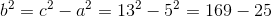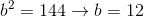### Example Question #3 : Calculating The Length Of The Side Of A Right Triangle

The hypotenuse of a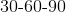triangle has length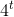. Which of the following is equal to the length of its shortest leg?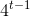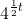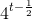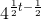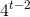Explanation:

The shortest leg of atriangle is one half the length of its hypotenuse. In this triangle, it is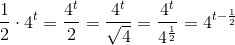Tired of practice problems?

Try live online GMAT Math prep today.